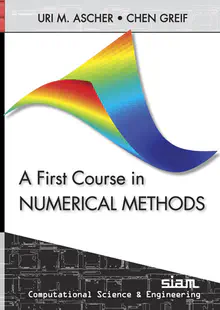# Math 614 Numerical Methods I

### Course Info

Almost everything is on the course canvas page.

### Responses to Feedback Sheet questions:

Questions in bold, answers in plain text.

#### Responses for Thursday February 2

• I think I would understand the properties of the norm and everything in today’s class if I saw more examples related to it! Here’s a nice example of a vector norm and here it is for matrix norms.
• Do we need to learn more about vector norms? A little bit, but I’ll save that for when we need them.
• A student did not understand the part during “matrix norms that went like $\lVert A \rVert_\infty = \max_i |{(Av)}_i|$. That’s just the definition, recall that we also need $\lVert v \rVert_\infty = \max_i |v_i| = 1$.
• What do equivalent norms mean in application? How should I understand it? Basically the important thing to realize that if two norms are equivalent, then small things in one norm are small in the other, and large things in one norm are large in the other. Therefore, when we want to show that our approximation error is small, any equivalent norm will do and we can use whichever one makes our calculation easiest.
• Why do people study norms on infinite dimensional vector spaces? Good question! Here’s one answer: a simple example would be the set of all bounded continuous functions. To make this even easier, suppose these are continuous functions on the domain $0<x<1$ such that $f(0)=f(1)=0$. This satisfies the definitions of a vector space (if $f(x)$ and $g(x)$ are in the space so is $h(x)=af(x)+b(g(x)$). Just like we want to know if a sequence of numbers converges to a limit, we may like to know if a sequence of functions converges to a limit. If we define $\lVert f \rVert = \max_{0<x<1}|f(x)|$, then this works.
• If $A = \begin{pmatrix} 1 & 2 & 3 \\ -4 & 5 &-6\end{pmatrix}$, why is $\lVert A \rVert_\infty = 15$ and $\lVert A \rVert_1 = 9$? Because the infinity-norm is the largest sum of the absolute values over any row ($4+5+6$) and the one-norm is the largest such column sum ($3+6$).
• For two norms $\lVert \cdot \rVert_a$ and $\lVert \cdot \rVert_b$, do the two positive constants $c_1$ and $c_2$ in the inequality $c_1\lVert v \rVert_a < \lVert v \rVert_b < c_2\lVert v \rVert_a$ have to satisfy $c_1<c_2$? Yes, of course.
• What is $\text{range}A$? This is just the set of all vectors $x$ such that $x = Av$ fro some other vector $v$. Another way to say this is that $v$ is a linear combination of the columns of $A$.
• What is the spectral decomposition?

Here’s what the textbook has to say

Spectral decomposition: Let $X$ be the matrix whose columns are eigenvectors of $A$, and suppose that $X$ is square and nonsingular. Then \begin{aligned} A X & =A\left[\mathbf{x}_1, \mathbf{x}_2, \ldots, \mathbf{x}_n\right] \ & =\left[\lambda_1 \mathbf{x}_1, \lambda_2 \mathbf{x}_2, \ldots, \lambda_n \mathbf{x}_n\right] \ & =X \Lambda \end{aligned} where $\Lambda$ is a diagonal matrix with the eigenvalues on its diagonal, $\Lambda=\operatorname{diag}\left(\lambda_1, \lambda_2\right.$, $\left.\ldots, \lambda_n\right)$. Therefore, we can write $$A=X \Lambda X^{-1} \text {. }$$